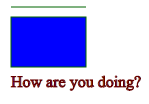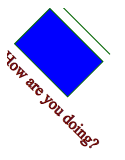# Introduction to SVG Part - 1SVG Elements

Introduction

SVG is short for Scalable Vector Graphics. It is a graphic format in which the shapes are specified in XML. The XML is then rendered by an SVG viewer. SVG graphics do NOT lose any quality if they are zoomed or re-sized.

Usage

SVG is mostly useful for vector type diagrams like:

• Two-dimensional graphs in an X,Y coordinate system.
• Column charts, pie charts etc.
• Scalable icons and logos for web, tablet and mobile apps and webapps.
• Architecture and design diagrams

It is possible to modify SVG images generated in the browser via JavaScript. This makes it possible to use SVG for more dynamic presentations, and even small games

For example:

Following example shows a simple rectangle with 50 x 50 pixels inside HTML page. The stroke color (the rectangle border) is set to the HTML color #ff0000. The fill color is set to #0000ff. The stroke-width is for setting border width.

<svg>

<rect x="10" y="10" height="50" width="50" stroke-width="2"

style="stroke:#ff0000; fill: #0000ff"/>

</svg>SVG has some predefined shapes elements developer can use them to build different shapes, some of the predefined shapes are:

• Circle – <circle>
• Ellipse – <ellipse>
• Line – <line>
• Polygon – <polygon>
• Polyline – <polyline>
• Path – <path>

SVG element uses coordinate system

In normal Cartesian coordinate system the point x=0, y=0 is at the bottom left corner of the graph but in the SVG coordinate system its top left corner.

For example:

<svg x="0" y="0">

<rect x="0" y="0" height="50" width="50" stroke-width="2"

style="stroke:#ff0000; fill: #0000ff"/>

</svg>

Now try to change value of x and y for both <svg> and <rect>, you can see the position of rectangle is also changing.

SVG elements can be nested inside each other

For example:

<svg>

<svg x="10">

<rect x="10" y="10" height="100" width="100"

style="stroke:#ff0000; fill: #0000ff"/>

</svg>

<svg x="200">

<rect x="10" y="10" height="100" width="100"

style="stroke:#009900; fill: #00cc00"/>

</svg>

</svg>

Nesting SVG elements can be useful to group SVG shapes together, and position them as a collection. All shapes nested inside svg element will be positioned (x, y) relative to the position (x, y) of its enclosing svg element. By moving the x and y coordinates of the enclosing svg element, you move all the nested shapes too.

To avoid this SVG nesting SVG g element is used to group SVG shapes together.

SVG g element

Once grouped you can transform the whole group of shapes as if it was a single shape.

For example:

<svg>

<g>

<line x1="10" y1="10" x2="85" y2="10" style="stroke: #006600;"/>

<rect x="10" y="20" height="50" width="75"

style="stroke: #006600; fill: #0000ff"/>

<text x="10" y="90" style="stroke: #660000; fill: #660000">

How are you doing?

</text>

</g>

</svg>

Resulting image:This example shows grouping of three shapes in a g element. Now let’s try to rotate the g element.

For example:

<svg>

<g transform="rotate(45 50 50)">

<line x1="10" y1="10" x2="85" y2="10" style="stroke: #006600;"/>

<rect x="10" y="20" height="50" width="75"

style="stroke: #006600; fill: #0000ff"/>

<text x="10" y="90" style="stroke: #660000; fill: #660000">

How are you doing?

</text>

</g>

</svg>

Resulting Image:Here you can see that all shapes within the <g>-element are rotated 45 degrees around the point 50, 50.

As you can see the image is getting cut, this is the drawback of g element as it’s not having X and Y attributes.

You cannot move the <g>-element including all nested shapes around, just by changing the <g>-element’s x and y attributes.

To move the contents of a <g>-element you can only do so using the transform attribute, using the “translate” function, like this: transform=”translate(x,y)”.

For example:

<svg width="200" height="200">

<g transform="translate(20, 20)">

<line x1="10" y1="10" x2="85" y2="10" style="stroke: #006600;"/>

<rect x="10" y="20" height="50" width="75"

style="stroke: #006600; fill: #0000ff"/>

<text x="10" y="90" style="stroke: #660000; fill: #660000">

How are you doing?

</text>

</g>

</svg>

Now try to change the position of g by changing the value of translate. This way you can decide where to set the g element inside svg.

More information on SVG element will see in the next blog, information like Viewport and Viewbox of SVG